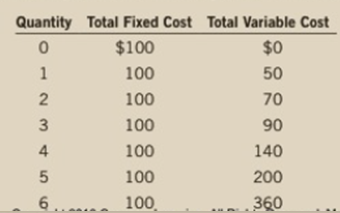Chapter 14, Problem 5PA

Chapter
Section
Textbook Problem

Ball Bearings, Inc., faces costs of production as follows:a. Calculate the company’s average fixed cost, average variable cost, average total cost, and marginal cost at each level of production.b. The price of a case of ball bearings is $50. Seeing that he can’t make a profit, the chief executive officer (CEO) decides to shut down operations. What is the firm’s profit/loss? Was this a wise decision? Explain.c. Vaguely remembering his introductory economics course, the chief financial officer tells the CEO it is better to produce 1 case of ball bearings, because marginal revenue equals marginal cost at that quantity. What is the firm’s profit/loss at that level of production? Was this the best decision? Explain. Subpart (a): To determine The fixed cost, average variable cost, average total cost, and marginal cost, profit or loss of the firm if they shutdown, profit or loss of the firm if they continue to produce. Explanation The table – 1 represents the value of the cost of the production. Table – 1  Quantity Total fixed cost Total variable cost 0 100 0 1 100 50 2 100 70 3 100 90 4 100 140 5 100 200 6 100 360 The average fixed cost can be determined by using the formula. Average fixed cost=Total fixed costQuantity (1) Substitute the respective value in equation (1) to calculate the average fixed cost for 1st unit. Average fixed cost=1001=100 The average fixed cost is$100.

The table - 2 shows the value of the average fixed cost that is obtained by using the equation (1).

Table - 2

 Quantity Total fixed cost Total variable cost Average fixed cost 0 100 0 – 1 100 50 100 2 100 70 50 3 100 90 33.3 4 100 140 25 5 100 200 20 6 100 360 16.7

The average variable cost can be determined by using the formula.

Average variable cost=Total variable costQuantity (2)

Substitute the respective value in equation (2) to calculate the average variable cost for 1st unit.

Average variable cost=501=50

The average variable cost is \$50.

The table -3 shows the value of the average variable cost that is obtained by using the equation (2).

Table – 3

 Quantity Total fixed cost Total variable cost Average fixed cost Average variable cost 0 100 0 – – 1 100 50 100 50 2 100 70 50 35 3 100 90 33.3 30 4 100 140 25 35 5 100 200 20 40 6 100 360 16.7 60

The average total cost can be determined by using the following formula.

Average total cost=Average variable cost +Average fixed cost (3)

Substitute the respective value in equation (3) to calculate the average total cost for 1st unit

Subpart (b):

To determine
The fixed cost, average variable cost, average total cost, and marginal cost, profit or loss of the firm if they shutdown, profit or loss of the firm if they continue to produce.

Subpart (c):

To determine
The fixed cost, average variable cost, average total cost, and marginal cost, profit or loss of the firm if they shutdown, profit or loss of the firm if they continue to produce.

Still sussing out bartleby?

Check out a sample textbook solution.

See a sample solution

The Solution to Your Study Problems

Bartleby provides explanations to thousands of textbook problems written by our experts, many with advanced degrees!

Get Started

Find more solutions based on key concepts